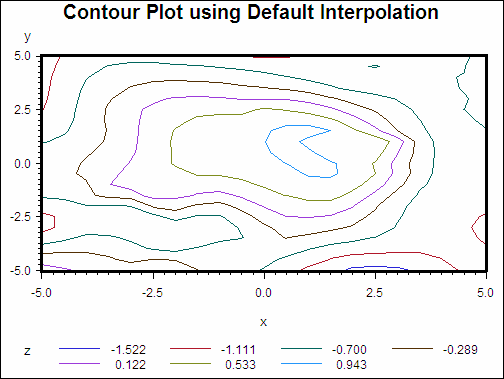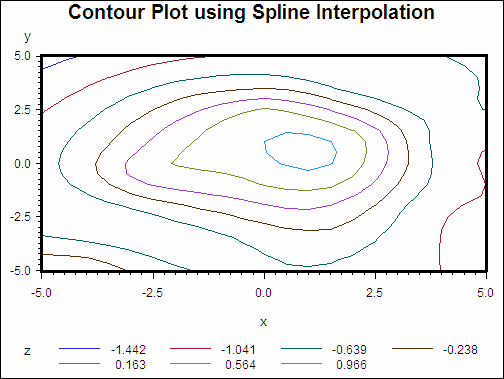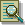The G3GRID Procedure

# Example 4: Spline Interpolation

Procedure features:

GRID statement options:

 AXIS1= AXIS2= SPLINE
Data set: NUMS (see Using the Default Interpolation Method)
Sample library member: GTGSPLIN

This example demonstrates the default interpolation method when used by the GCONTOUR procedure to generate a contour plot from the resulting output data set.

Contour Plot Using Default Interpolation (gtgsplin)The second plot, demonstrates the spline interpolation method when used by the GCONTOUR procedure to generate a contour plot from the resulting output data set.

Contour Plot Using Spline Interpolation (gtgsplin)`goptions reset=all border;``title "Contour Plot using Default Interpolation";``axis1 width=3;````proc g3grid data=nums out=numdef; grid y*x=z / axis1=-5 to 5 by .5 axis2=-5 to 5 by .5; run;``````proc gcontour data=numdef; plot y*x=z / haxis=axis1 vaxis=axis1; run; quit;````title "Contour Plot using Spline Interpolation";````proc g3grid data=nums out=numspl; grid y*x=z / spline axis1=-5 to 5 by .5 axis2=-5 to 5 by .5; run;``````proc gcontour data=numspl; plot y*x=z / haxis=axis1 vaxis=axis1; run; quit;```Previous Page | Next Page | Top of Page